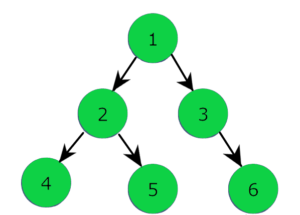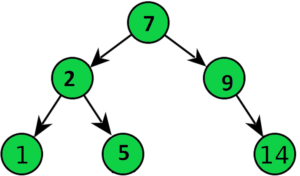# Difference between Binary Tree and Binary Search Tree

In this article, we will look at the difference between Binary Tree and Binary Search Tree. These topics are very important because these act as an underlying data structure for various other data structures. So, we will look at the description of each with examples and compare their properties.

### Binary Tree

A Binary tree is a type of Tree data structure, Non-Linear (data is not contiguous or in sequence) in nature. It represents data in a hierarchical format. A binary tree like a regular tree comprises of nodes that are not Ordered. Each node in a binary tree can have a maximum of 2 child nodes. It can have 0,1 or 2 nodes. A node is represented as a parent or child node. A Parent node can have at most two children- Left Child and Right Child. The node which has at least two children is called Internal Node. Hence, each node in memory contains the following attributes:

• Data (Can be of any type).
• Left Pointer which has the reference for Left Child.
• Right Pointer which has the reference for Right Child.

Let us look at the following example:In the above binary tree, you can see the tree is not ordered, the Node 1 is the root node. The left arrow and the right arrow indicate the left and right child of each node respectively. Nodes present at the last level are the leaf nodes. Hence, the given binary tree has Nodes 1, 2, 3 as the parent nodes. Node 1 and Node 2 are the internal nodes as they have 2 children.

#### Operations on Binary Tree with Complexities:

• Search: To search an element we need to traverse all nodes in the tree. With the assumption that we do Level Order Traversal for searching the time complexity to implement search is O(n) for n nodes in a tree.
• Insert: If we consider a Skewed Binary Tree, then inserting an element would require traversing to the last node of the tree then insert it. So the overall complexity will be O(n).
• Delete: For deleting a node we have to first search it in the tree then deallocate the memory. Thus, like the search operation, it also requires O(n) time.

### Binary Search Tree

A Binary Search Tree (BST) is a special type of binary tree. It is a node based binary tree data structure where the nodes are arranged in a specific order. The nodes contain the same structure as in a binary tree but they differ in arrangements. It is an Ordered tree, which follows the given conditions:

• The left child of a node contains value or data less than it’s parent node.
• The right child of a node contains value or data greater than the parent node’s data.
• Hence, the left subtrees contain nodes with values lesser than the root of the tree and the right subtrees contain nodes with values greater than root of the tree.
• The left and the right subtrees of each node if they exist must also be a binary search tree and should follow the above rules. As each node’s data must be greater or lesser than it’s parent node so no nodes with Duplicate keys or values are allowed.

Below, a typical Binary Search Tree is shown:In the above tree, you can see the tree is ordered. For every parent node, e.g. Node 7 (Root Node), it’s left child (Node 2) is smaller than it whereas it’s right child (Node 9) is greater than it. Each subtree of a node is itself  a binary search tree and follows the above rules.

Note: The In-Order traversal of the binary search tree will give the nodes in sorted order. The leftmost node has the smallest node value.

#### Operations on BST with Complexities:

The whole idea of using a BST or Binary Search Tree is to optimize the search operation for each lookup. If we search a Node in a BST we remove half sub-tree at every step because of it’s Ordered nature. For instance, consider a BST of n nodes, at each comparison we search for a node at either the left half or the right half. We reduce the search space to n/2 at each step until the element is found. The worst case for operations occurs in Skewed Binary Search Trees.

• Search: Searching for an element in a binary search tree generally takes O(log n) time or O(h), h is the height of the tree. In the worst case, the time taken to search an element is O(n) if the given BST is Skewed BST.
• Insert: The time is the same as search operation O(log n) or O(h) but in the worst case, it takes O(n) time.
• Delete: Overall complexity to search and deallocate memory is same as above O(log n) but in worst case O(n) time.

Now Let us look at the key differences between Binary Tree and a BST:

## Binary Tree vs Binary Search Tree

 Binary Tree Binary Search Tree 1. It is a Non-linear data structure, a specialized form of Tree data structure, where each node has a maximum of two child nodes. 1. It is a node based binary tree where each node has maximum of two children and the trees on the left half and right half of each node are itself a Binary Search Tree. 2. There is no Ordering of data while arranging nodes in a binary tree. 2. A BST is an Ordered tree, the value of the left child is smaller than it’s parent node and the value of a right child is greater than the value of it’s parent node. Hence, The same is followed for the subtrees. 3. It is useful in representing data in a Hierarchical format. 3. It is useful in representing data in an Ordered format. 4. Nodes with Duplicate values are allowed. 4. Duplicate nodes are not allowed. 5. The operations on a binary tree take a comparatively longer time. As a result the Search, Insert, and Delete operation takes O(n) time. 5. Binary Search Trees are sorted which provides fast search, insert and delete operation taking almost O(log n) time. Hence, for lookups we mainly use BST’s as all the keys are arranged in sorted order. 6. It acts as the base for implementing Full Binary Tree, BST’s, Perfect Binary Tree, etc. 6. It is used in implementing Balanced Binary Search Trees like AVL Trees, Red Black Trees, etc.

That’s it for the article we looked at them in detail description of each with their examples along with the major differences.

Feel free to ask your doubts or to leave suggestions in the comment section below.

### 1 thought on “Difference between Binary Tree and Binary Search Tree”

1.BinaryCalculators